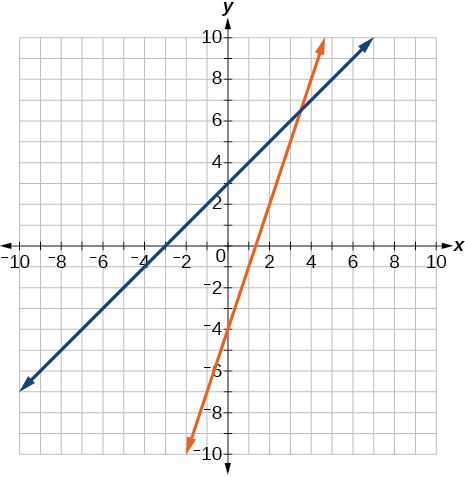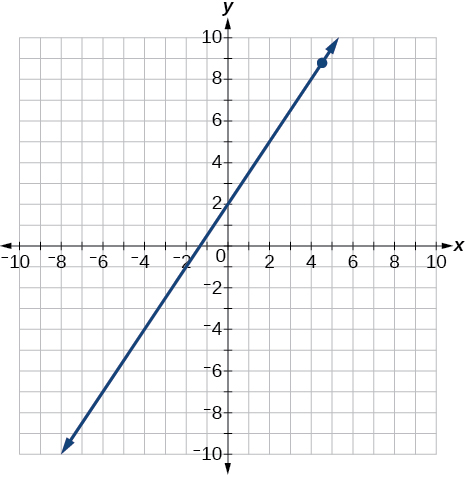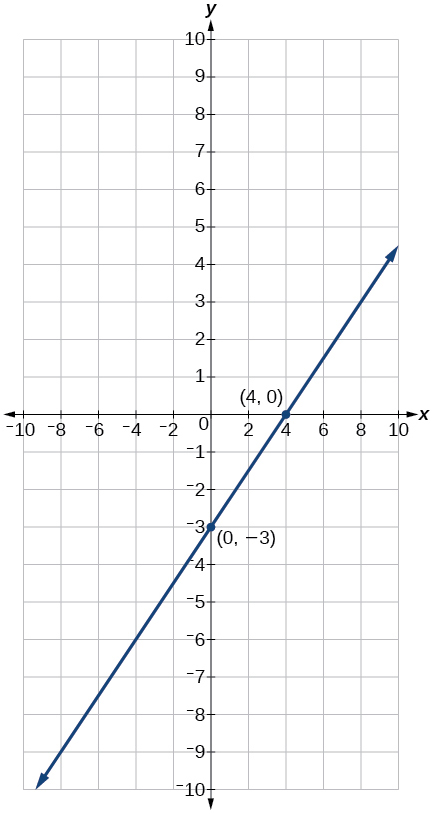# 2.7 Linear inequalities and absolute value inequalities  (Page 7/11)

 Page 7 / 11

A man has 72 ft. of fencing to put around a rectangular garden. If the length is 3 times the width, find the dimensions of his garden.

A truck rental is $25 plus$.30/mi. Find out how many miles Ken traveled if his bill was \$50.20.

84 mi

## Complex Numbers

For the following exercises, use the quadratic equation to solve.

${x}^{2}-5x+9=0$

$2{x}^{2}+3x+7=0$

$x=\frac{-3}{4}±\frac{i\sqrt{47}}{4}$

For the following exercises, name the horizontal component and the vertical component.

$4-3i$

$-2-i$

horizontal component $\text{\hspace{0.17em}}-2;$ vertical component $\text{\hspace{0.17em}}-1$

For the following exercises, perform the operations indicated.

$\left(9-i\right)-\left(4-7i\right)$

$\left(2+3i\right)-\left(-5-8i\right)$

$7+11i$

$2\sqrt{-75}+3\sqrt{25}$

$\sqrt{-16}+4\sqrt{-9}$

$16i$

$-6i\left(i-5\right)$

${\left(3-5i\right)}^{2}$

$-16-30i$

$\sqrt{-4}·\sqrt{-12}$

$\sqrt{-2}\left(\sqrt{-8}-\sqrt{5}\right)$

$-4-i\sqrt{10}$

$\frac{2}{5-3i}$

$\frac{3+7i}{i}$

$x=7-3i$

For the following exercises, solve the quadratic equation by factoring.

$2{x}^{2}-7x-4=0$

$3{x}^{2}+18x+15=0$

$x=-1,-5$

$x=0,\frac{9}{7}$

For the following exercises, solve the quadratic equation by using the square-root property.

${x}^{2}=49$

${\left(x-4\right)}^{2}=36$

$x=10,-2$

For the following exercises, solve the quadratic equation by completing the square.

${x}^{2}+8x-5=0$

$4{x}^{2}+2x-1=0$

$x=\frac{-1±\sqrt{5}}{4}$

For the following exercises, solve the quadratic equation by using the quadratic formula. If the solutions are not real, state No real solution .

$2{x}^{2}-5x+1=0$

$15{x}^{2}-x-2=0$

$x=\frac{2}{5},\frac{-1}{3}$

For the following exercises, solve the quadratic equation by the method of your choice.

${\left(x-2\right)}^{2}=16$

${x}^{2}=10x+3$

$x=5±2\sqrt{7}$

## Other Types of Equations

For the following exercises, solve the equations.

${x}^{\frac{3}{2}}=27$

${x}^{\frac{1}{2}}-4{x}^{\frac{1}{4}}=0$

$x=0,256$

$4{x}^{3}+8{x}^{2}-9x-18=0$

$3{x}^{5}-6{x}^{3}=0$

$x=0,±\sqrt{2}$

$\sqrt{x+9}=x-3$

$\sqrt{3x+7}+\sqrt{x+2}=1$

$x=-2$

$|3x-7|=5$

$|2x+3|-5=9$

$x=\frac{11}{2},\frac{-17}{2}$

## Linear Inequalities and Absolute Value Inequalities

For the following exercises, solve the inequality. Write your final answer in interval notation.

$5x-8\le 12$

$-2x+5>x-7$

$\left(-\infty ,4\right)$

$\frac{x-1}{3}+\frac{x+2}{5}\le \frac{3}{5}$

$|3x+2|+1\le 9$

$\left[\frac{-10}{3},2\right]$

$|5x-1|>14$

$|x-3|<-4$

No solution

For the following exercises, solve the compound inequality. Write your answer in interval notation.

$-4<3x+2\le 18$

$3y<1-2y<5+y$

$\left(-\frac{4}{3},\frac{1}{5}\right)$

For the following exercises, graph as described.

Graph the absolute value function and graph the constant function. Observe the points of intersection and shade the x -axis representing the solution set to the inequality. Show your graph and write your final answer in interval notation.

$|x+3|\ge 5$

Graph both straight lines (left-hand side being y1 and right-hand side being y2) on the same axes. Find the point of intersection and solve the inequality by observing where it is true comparing the y -values of the lines. See the interval where the inequality is true.

$x+3<3x-4$

Where the blue is below the orange line; point of intersection is $\text{\hspace{0.17em}}x=3.5.$

$\left(3.5,\infty \right)$## Chapter practice test

Graph the following: $\text{\hspace{0.17em}}2y=3x+4.$

$y=\frac{3}{2}x+2$

x y
0 2
2 5
4 8Find the x- and y -intercepts for the following:

$2x-5y=6$

Find the x- and y -intercepts of this equation, and sketch the graph of the line using just the intercepts plotted.

$3x-4y=12$

$\left(0,-3\right)$ $\left(4,0\right)$Find the exact distance between $\text{\hspace{0.17em}}\left(5,-3\right)\text{\hspace{0.17em}}$ and $\text{\hspace{0.17em}}\left(-2,8\right).\text{\hspace{0.17em}}$ Find the coordinates of the midpoint of the line segment joining the two points.

Write the interval notation for the set of numbers represented by $\text{\hspace{0.17em}}\left\{x|x\le 9\right\}.$

$\left(-\infty ,9\right]$

Solve for x : $\text{\hspace{0.17em}}5x+8=3x-10.$

Solve for x : $\text{\hspace{0.17em}}3\left(2x-5\right)-3\left(x-7\right)=2x-9.$

$x=-15$

Solve for x : $\text{\hspace{0.17em}}\frac{x}{2}+1=\frac{4}{x}$

Solve for x : $\text{\hspace{0.17em}}\frac{5}{x+4}=4+\frac{3}{x-2}.$

$x\ne -4,2;$ $x=\frac{-5}{2},1$

The perimeter of a triangle is 30 in. The longest side is 2 less than 3 times the shortest side and the other side is 2 more than twice the shortest side. Find the length of each side.

Solve for x . Write the answer in simplest radical form.

$\frac{{x}^{2}}{3}-x=\frac{-1}{2}$

$x=\frac{3±\sqrt{3}}{2}$

Solve: $\text{\hspace{0.17em}}3x-8\le 4.$

Solve: $\text{\hspace{0.17em}}|2x+3|<5.$

$\left(-4,1\right)$

Solve: $\text{\hspace{0.17em}}|3x-2|\ge 4.$

For the following exercises, find the equation of the line with the given information.

Passes through the points $\text{\hspace{0.17em}}\left(-4,2\right)\text{\hspace{0.17em}}$ and $\text{\hspace{0.17em}}\left(5,-3\right).$

$y=\frac{-5}{9}x-\frac{2}{9}$

Has an undefined slope and passes through the point $\text{\hspace{0.17em}}\left(4,3\right).$

Passes through the point $\text{\hspace{0.17em}}\left(2,1\right)\text{\hspace{0.17em}}$ and is perpendicular to $\text{\hspace{0.17em}}y=\frac{-2}{5}x+3.$

$y=\frac{5}{2}x-4$

Add these complex numbers: $\text{\hspace{0.17em}}\left(3-2i\right)+\left(4-i\right).$

Simplify: $\text{\hspace{0.17em}}\sqrt{-4}+3\sqrt{-16}.$

$14i$

Multiply: $\text{\hspace{0.17em}}5i\left(5-3i\right).$

Divide: $\text{\hspace{0.17em}}\frac{4-i}{2+3i}.$

$\frac{5}{13}-\frac{14}{13}i$

Solve this quadratic equation and write the two complex roots in $\text{\hspace{0.17em}}a+bi\text{\hspace{0.17em}}$ form: $\text{\hspace{0.17em}}{x}^{2}-4x+7=0.$

Solve: $\text{\hspace{0.17em}}{\left(3x-1\right)}^{2}-1=24.$

$x=2,\frac{-4}{3}$

Solve: $\text{\hspace{0.17em}}{x}^{2}-6x=13.$

Solve: $\text{\hspace{0.17em}}4{x}^{2}-4x-1=0$

$x=\frac{1}{2}±\frac{\sqrt{2}}{2}$

Solve:

$\sqrt{x-7}=x-7$

Solve: $\text{\hspace{0.17em}}2+\sqrt{12-2x}=x$

$4$

Solve: $\text{\hspace{0.17em}}{\left(x-1\right)}^{\frac{2}{3}}=9$

For the following exercises, find the real solutions of each equation by factoring.

$2{x}^{3}-{x}^{2}-8x+4=0$

$x=\frac{1}{2},2,-2$

${\left(x+5\right)}^{2}-3\left(x+5\right)-4=0$

#### Questions & Answers

write down the polynomial function with root 1/3,2,-3 with solution
if A and B are subspaces of V prove that (A+B)/B=A/(A-B)
write down the value of each of the following in surd form a)cos(-65°) b)sin(-180°)c)tan(225°)d)tan(135°)
Prove that (sinA/1-cosA - 1-cosA/sinA) (cosA/1-sinA - 1-sinA/cosA) = 4
what is the answer to dividing negative index
In a triangle ABC prove that. (b+c)cosA+(c+a)cosB+(a+b)cisC=a+b+c.
give me the waec 2019 questions
the polar co-ordinate of the point (-1, -1)
prove the identites sin x ( 1+ tan x )+ cos x ( 1+ cot x )= sec x + cosec x
tanh`(x-iy) =A+iB, find A and B
B=Ai-itan(hx-hiy)
Rukmini
what is the addition of 101011 with 101010
If those numbers are binary, it's 1010101. If they are base 10, it's 202021.
Jack
extra power 4 minus 5 x cube + 7 x square minus 5 x + 1 equal to zero
the gradient function of a curve is 2x+4 and the curve passes through point (1,4) find the equation of the curve
1+cos²A/cos²A=2cosec²A-1
test for convergence the series 1+x/2+2!/9x3ByByBy David MartinByByByBy Lakeima Roberts